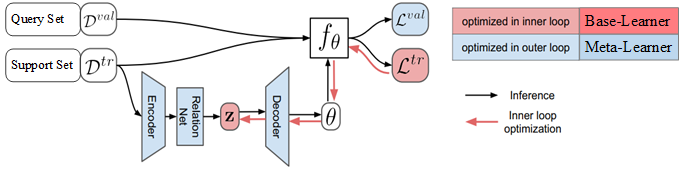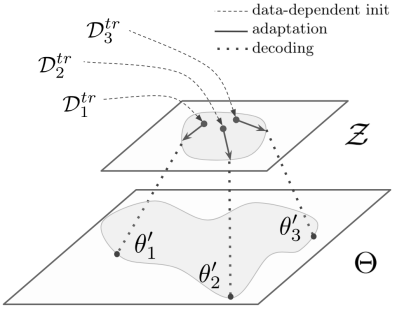# Latent Embedding Optimization¶

Latent Embedding Optimization (LEO) 学习模型参数的低维潜在嵌入，并在这个低维潜在空间中执行基于优化的元学习，将基于梯度的自适应过程与模型参数的基础高维空间分离。

## 1 LEO¶LEO 包括基础学习器和元学习器，还包括编码器和解码器。 在基础学习器中，编码器将高维输入数据映射成特征向量， 解码器将输入数据的特征向量映射成输入数据属于各个类别的概率值， 基础学习器使用元学习器提供的元参数进行参数更新，给出数据标注的预测结果。 元学习器为基础学习器的编码器和解码器提供元参数， 元参数包括特征提取模型的参数、编码器的参数、解码器的参数等， 通过最小化所有任务上的泛化误差，更新元参数。

## 2 基础学习器¶

### 2.1 编码器¶

$$n$$ 个类别的输入数据的特征记为 $$z_{n}$$ 。 对于输入数据，首先，使用编码器 $$g_{\phi_{e}}$$ 对属于第 $$n$$ 个类别的输入数据进行特征提取； 然后，使用关系网络 $$g_{\phi_r}$$ 计算特征之间的距离， 综合考虑训练集中所有样本点之间的距离，计算这些距离的平均值和离散程度； 第 $$n$$ 个类别输入数据的特征 $$z_{n}$$ 服从高斯分布， 且高斯分布的期望是这些距离的平均值，高斯分布的方差是这些距离的离散程度， 具体的计算公式如下：

\begin{split} \begin{aligned} &\mu_{n}^{e}, \sigma_{n}^{e}=\frac{1}{N K^{2}} \sum_{k_{n}=1}^{K} \sum_{m=1}^{N} \sum_{k_{m}=1}^{K} g_{\phi_{r}}\left[g_{\phi_{e}}\left(x_{n}^{k_{n}}\right), g_{\phi_{e}}\left(x_{m}^{k_{m}}\right)\right] \\ &z_{n} \sim q\left(z_{n} \mid D_{n}^{\mathrm{tr}}\right)=N\left\{\mu_{n}^{e}, \operatorname{diag}\left(\sigma_{n}^{e}\right)^{2}\right\} \end{aligned} \end{split}

### 2.2 解码器¶

\begin{split} \begin{aligned} &\mu_{n}^{d}, \sigma_{n}^{d}=g_{\phi_{d}}\left(z_{n}\right) \\ &w_{n} \sim q\left(w \mid z_{n}\right)=N\left\{\mu_{n}^{d}, \operatorname{diag}\left(\sigma_{n}^{d}\right)^{2}\right\} \end{aligned} \end{split}### 2.3 基础学习器更新过程¶

$L_{\varepsilon}^{\mathrm{tr}}\left(f_{\theta_{\varepsilon}}\right)=\sum_{(x, y) \in D_{\varepsilon}^{\mathrm{tr}}}\left[-w_{y} \boldsymbol{x}+\log \sum_{j=1}^{N} \mathrm{e}^{w_{j} x}\right]$

$z_{n}^{\prime}=z_{n}-\alpha \nabla_{z_{n}} L_{\varepsilon}^{t r}\left(f_{\theta_{\varepsilon}}\right),$

## 3 元学习器更新过程¶

$\min _{\phi_{e}, \phi_{r}, \phi_{d}} \sum_{\varepsilon}\left[L_{\varepsilon}^{\mathrm{val}}\left(f_{\theta_{\varepsilon}^{\prime}}\right)+\beta D_{\mathrm{KL}}\left\{q\left(z_{n} \mid {D}_{n}^{\mathrm{tr}}\right) \| p\left(z_{n}\right)\right\}+\gamma\left\|s\left(\boldsymbol{z}_{n}^{\prime}\right)-\boldsymbol{z}_{n}\right\|_{2}^{2}\right]+R$

$R=\lambda_{1}\left(\left\|\phi_{e}\right\|_{2}^{2}+\left\|\phi_{r}\right\|_{2}^{2}+\left\|\phi_{d}\right\|_{2}^{2}\right)+\lambda_{2}\left\|C_{d}-\mathbb{I}\right\|_{2}$

## 4 LEO 算法流程¶

LEO 算法流程

1. randomly initialize $$\phi_{e}, \phi_{r}, \phi_{d}$$

2. let $$\phi=\left\{\phi_{e}, \phi_{r}, \phi_{d}, \alpha\right\}$$

3. while not converged do:

1. for number of tasks in batch do:

1. sample task instance $$\mathcal{T}_{i} \sim \mathcal{S}^{t r}$$

2. let $$\left(\mathcal{D}^{t r}, \mathcal{D}^{v a l}\right)=\mathcal{T}_{i}$$

3. encode $$\mathcal{D}^{t r}$$ to z using $$g_{\phi_{e}}$$ and $$g_{\phi_{r}}$$

4. decode $$\mathbf{z}$$ to initial params $$\theta_{i}$$ using $$g_{\phi_{d}}$$

5. initialize $$\mathbf{z}^{\prime}=\mathbf{z}, \theta_{i}^{\prime}=\theta_{i}$$

6. for number of adaptation steps do:

1. compute training loss $$\mathcal{L}_{\mathcal{T}_{i}}^{t r}\left(f_{\theta_{i}^{\prime}}\right)$$

2. perform gradient step w.r.t. $$\mathbf{z}^{\prime}$$:

3. $$\mathbf{z}^{\prime} \leftarrow \mathbf{z}^{\prime}-\alpha \nabla_{\mathbf{z}^{\prime}} \mathcal{L}_{\mathcal{T}_{i}}^{t r}\left(f_{\theta_{i}^{\prime}}\right)$$

4. decode $$\mathbf{z}^{\prime}$$ to obtain $$\theta_{i}^{\prime}$$ using $$g_{\phi_{d}}$$

7. end for

8. compute validation loss $$\mathcal{L}_{\mathcal{T}_{i}}^{v a l}\left(f_{\theta_{i}^{\prime}}\right)$$

2. end for

3. perform gradient step w.r.t $$\phi$$$$\phi \leftarrow \phi-\eta \nabla_{\phi} \sum_{\mathcal{T}_{i}} \mathcal{L}_{\mathcal{T}_{i}}^{v a l}\left(f_{\theta_{i}^{\prime}}\right)$$

4. end while

(1) 初始化元参数：编码器参数 $$\phi_{e}$$、关系网络参数 $$\phi_{r}$$、解码器参数 $$\phi_{d}$$, 在元学习器中更新的元参数包括 $$\phi=\left\{\phi_e, \phi_r,\phi_d \right\}$$

(2) 使用片段式训练模式， 随机抽取任务 $$\varepsilon$$, $${D}_{\varepsilon}^{\mathrm{tr}}$$ 是任务 $$\varepsilon$$ 的训练集， $${D}_{\varepsilon}^{\mathrm{val}}$$ 是任务 $$\varepsilon$$ 的验证集。

(3) 使用编码器 $$g_{\phi_{e}}$$ 和关系网络 $$g_{\phi_{r}}$$ 将任务 $$\varepsilon$$ 的训练集 $$D_{\varepsilon}^{\mathrm{tr}}$$ 编码成特征向量 $$z$$， 使用 解码器 $$g_{\phi_{d}}$$ 从特征向量映射到任务 $$\varepsilon$$ 的基础学习器参数 $${\theta}_{\varepsilon}$$， 基础学习器参数指的是输入数据属于每个类别的概率值向量； 计算任务 $$\varepsilon$$ 的训练集的损失函数 $$L_{\varepsilon}^{\mathrm{tr}}\left(f_{\theta_{\varepsilon}}\right)$$， 最小化任务 $$\varepsilon$$ 的损失函数，更新每个类别的特征向量：

$z_{n}^{\prime}=z_{n}-\alpha \nabla_{z_{n}} L_{\varepsilon}^{\mathrm{tr}}\left(f_{\theta_{\varepsilon}}\right)$

(4) 更新元参数, $$\phi \leftarrow \phi-\eta \nabla_{\phi} \sum_{\varepsilon} L_{\varepsilon}^{\text {val}}\left(f_{\theta_{\varepsilon}^{\prime}}\right)$$， 最小化所有任务 $$\varepsilon$$ 的验证集的损失和， 将更新后的元参数输人基础学习器，继续处理新的分类任务。

## 5 LEO 模型结构¶

LEO 是一种与模型无关的元学习， 中给出的各部分模型结构及参数如表1所示。

Part of the model

Architecture

Hiddenlayer

Shape of the output

Inference model ($$f_{\theta}$$)

3-layer MLP with ReLU

40

(12, 5, 1)

Encoder

3-layer MLP with ReLU

16

(12, 5, 16)

Relation Network

3-layer MLP with ReLU

32

(12, $$2\times 16$$)

Decoder

3-layer MLP with ReLU

32

(12, $$2\times 1761$$)

## 6 LEO 分类结果¶

Model

5-way 1-shot

5-way 5-shot

Matching networks (Vinyals et al., 2016)

43.56 $$\pm$$ 0.84 $$\%$$

55.31 $$\pm$$ 0.73 $$\%$$

Meta-learner LSTM (Ravi & Larochelle, 2017)

43.44 $$\pm$$ 0.77 $$\%$$

60.60 $$\pm$$ 0.71 $$\%$$

MAML (Finn et al., 2017)

48.70 $$\pm$$ 1.84 $$\%$$

63.11 $$\pm$$ 0.92 $$\%$$

LLAMA (Grant et al., 2018)

49.40 $$\pm$$ 1.83 $$\%$$

REPTILE (Nichol & Schulman, 2018)

49.97 $$\pm$$ 0.32 $$\%$$

65.99 $$\pm$$ 0.58 $$\%$$

PLATIPUS (Finn et al., 2018)

50.13 $$\pm$$ 1.86 $$\%$$

Meta-SGD (our features)

54.24 $$\pm$$ 0.03 $$\%$$

70.86 $$\pm$$ 0.04 $$\%$$

SNAIL (Mishra et al., 2018)

55.71 $$\pm$$ 0.99 $$\%$$

68.88 $$\pm$$ 0.92 $$\%$$

(Gidaris & Komodakis, 2018)

56.20 $$\pm$$ 0.86 $$\%$$

73.00 $$\pm$$ 0.64 $$\%$$

(Bauer et al., 2017)

56.30 $$\pm$$ 0.40 $$\%$$

73.90 $$\pm$$ 0.30 $$\%$$

(Munkhdalai et al., 2017)

57.10 $$\pm$$ 0.70 $$\%$$

70.04 $$\pm$$ 0.63 $$\%$$

DEML+Meta-SGD (Zhou et al., 2018)

58.49 $$\pm$$ 0.91 $$\%$$

71.28 $$\pm$$ 0.69 $$\%$$

58.50 $$\pm$$ 0.30 $$\%$$

76.70 $$\pm$$ 0.30 $$\%$$

(Qiao et al., 2017)

59.60 $$\pm$$ 0.41 $$\%$$

73.74 $$\pm$$ 0.19 $$\%$$

LEO

61.76 $$\pm$$ 0.08 $$\%$$

77.59 $$\pm$$ 0.12 $$\%$$

Model

5-way 1-shot

5-way 5-shot

MAML (deeper net, evaluated in Liu et al. (2018))

51.67 $$\pm$$ 1.81 $$\%$$

70.30 $$\pm$$ 0.08 $$\%$$

Prototypical Nets (Ren et al., 2018)

53.31 $$\pm$$ 0.89 $$\%$$

72.69 $$\pm$$ 0.74 $$\%$$

Relation Net (evaluated in Liu et al. (2018))

54.48 $$\pm$$ 0.93 $$\%$$

71.32 $$\pm$$ 0.78 $$\%$$

Transductive Prop. Nets (Liu et al., 2018)

57.41 $$\pm$$ 0.94 $$\%$$

71.55 $$\pm$$ 0.74 $$\%$$

Meta-SGD (our features)

62.95 $$\pm$$ 0.03 $$\%$$

79.34 $$\pm$$ 0.06 $$\%$$

LEO

66.33 $$\pm$$ 0.05 $$\%$$

81.44 $$\pm$$ 0.09 $$\%$$

## 7 LEO 的优点¶

• 新任务的初始参数以训练数据为条件，这使得任务特定的适应起点成为可能。 通过将关系网络结合到编码器中，该初始化可以更好地考虑所有输入数据之间的联合关系。

• 通过在低维潜在空间中进行优化，该方法可以更有效地适应模型的行为。 此外，通过允许该过程是随机的，可以表达在少数数据状态中存在的不确定性和模糊性。